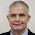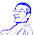## Friday, May 15, 2015

### How to correctly set color in the image() function?

Sometimes we want to make our own heatmap using image() function. I recently found it's tricky to set the color option there, as its manual has very little information on col:

I posted my question on BioStars. The short answer is: Unless the breaks is set, the range of Z is evenly cut into N intervals (where N = the length of color) and values in Z are assigned to the color of corresponding interval.

For example, when x=c(3,1,2,1) and col=c("blue","red",'green','yellow'), the minimal of x is assigned as the first color, and max to the last color. Any value between is calculated proportionally to a color. In this case, 2 is the the middle one, according to the principal that intervals are closed on the right and open on the left, it's assigned to "red". So, that's why we see the colors are yellow-->blue-->red-->blue.

```x=c(3,1,2,1)

image(1,1:length(x), matrix(x, nrow=1, ncol=length(x)), col=c("blue","red",'green','yellow'))```

In practice, unless we want to manually define the color break points, we just set the first and last color, it will automatically find colors for the values in Z.

collist<-c(0,1)
image(1:ncol(x),1:nrow(x), as.matrix(t(x)), col=collist, asp=1)

If we want to manually define the color break points, we need to

x=matrix(rnorm(100),nrow=10)*100
xmin=0; xmax=100;
x[x<xmin]=xmin; x[x>xmax]=xmax;
collist<-c("#053061","#2166AC","#4393C3","#92C5DE","#D1E5F0","#F7F7F7","#FDDBC7","#F4A582","#D6604D","#B2182B","#67001F")
ColorRamp<-colorRampPalette(collist)(10000)
ColorLevels<-seq(from=xmin, to=xmax, length=10000)
ColorRamp_ex <- ColorRamp[round(1+(min(x)-xmin)*10000/(xmax-xmin)) : round( (max(x)-xmin)*10000/(xmax-xmin) )]
par(mar=c(2,0,2,0), oma=c(3,3,3,3))
layout(matrix(seq(2),nrow=2,ncol=1),widths=c(1),heights=c(3,0.5))
image(t(as.matrix(x)), col=ColorRamp_ex, las=1, xlab="",ylab="",cex.axis=1,xaxt="n",yaxt="n")
image(as.matrix(ColorLevels),col=ColorRamp, xlab="",ylab="",cex.axis=1,xaxt="n",yaxt="n")
axis(1,seq(xmin,xmax,10),seq(xmin,xmax,10))

1.It one begins with

par(pty="s")

One gets the correct (square) aspect ratio

1.Thank you for the tips. I did not know it.

2.Nice description! The difficulties in making "image" plots were what led me and my co-author to write the `imagep()` and `colormap()` functions for the oce package. You can see some examples here:

http://dankelley.github.io/r/2014/04/30/colormap.html

With the colormap function you can define any of the color levels and breaks you want, as well as take advantage of a few predefined palettes (`oceColorsJet`, `oceColorsTwo`, oceColorsGebco`, etc). Then if you feed the return value from `colormap` into `imagep()`, you're guaranteed to get colors that actually match your data in the way you want.

1.Wow~ great tool! Thanks for letting me know. I will try it later.